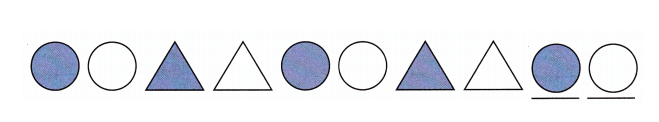Practice the questions of McGraw Hill Math Grade 4 Answer Key PDF Chapter 12 Test to secure good marks & knowledge in the exams.

Question 1.
What is a ray?
A ray is a part of a line that has 1 endpoint
It goes on without end in the other direction.

Question 2.
What is an angle?
An angle is a figure formed by two rays that share the same end point
The rays are the sides of angle
The end pint is the vertex of the angle.

Question 3.
Which pair of lines is parallel? Place a checkmark below the correct answer.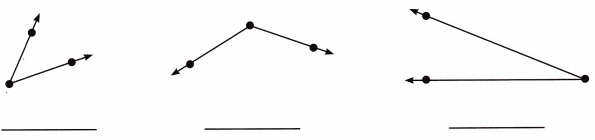There are no parallel lines in the given pairs of lines.

Question 4.
Which pair of lines is parallel? Place a checkmark below the correct answer.Explanation:
The first pair of lines are parallel lines
So, i placed a check mark under the first pair of lines.

Question 5.How many parallel lines does this shape have? ________
Does this shape have perpendicular lines? ________
Are all four sides the same length? ________
Name the shape. ________
The shape have 2 pars of parallel lines
Yes, the shape of perpendicular lines
No, opposite sides are same in length
The name of the shape is parallelogram.

Question 6.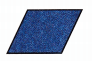How many parallel lines does this shape have? ________
Does this shape have perpendicular lines? ________
Are all four sides the same length? ________
Name the shape. ________
The shape have 2 pars of parallel lines
Yes, the shape of perpendicular lines
All the sides are same in length
The name of the shape is rhombus.

Classify each triangle as equilateral, scalene, or isosceles. Then classify each as acute, obtuse, or right.

Question 7.Right
Scalene

Explanation:
The triangle is right and scalene.

Question 8.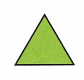Acute
Equilateral

Explanation:
The triangle is an acute and equilateral.

Question 9.Acute
Scalene

Explanation:
The triangle is an acute and scalene.

Question 10.
Draw a line segment. Label it $$\overline{A B}$$.Explanation:
I drew a line segment and labeled it $$\overline{A B}$$.

Question 11.
Draw an obtuse angle. Label it ∠RPQ.Explanation:
I drew an obtuse angle triangle and labeled it ∠RPQ.

Question 12.
Draw a pair of perpendicular lines. Label them $$\overleftrightarrow{M N}$$ and $$\overleftrightarrow{O P}$$.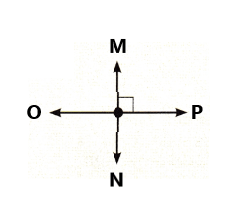Explanation:
I drew a pair of perpendicular lines and labeled the $$\overleftrightarrow{M N}$$ and $$\overleftrightarrow{O P}$$.

Draw lines of symmetry on each shape if you can.

Question 13.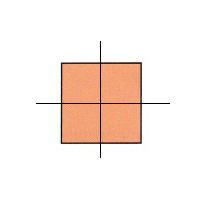Explanation:
I drew 2 lines of symmetry in the given shape.

Question 14.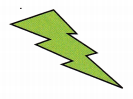The above given figure cannot be folded into two equal parts
So, it do not have any lines of symmetry.

Question 15.
Jenny draws this pattern.What shapes will complete the pattern?